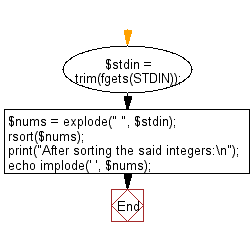﻿ PHP Exercise: Accepts six numbers as input and sorts them in descending order - w3resource

# PHP Exercises: Accepts six numbers as input and sorts them in descending order

## PHP: Exercise-55 with Solution

Write a PHP program that accepts six numbers as input and sorts them in descending order.

Input: Input consists of six numbers n1, n2, n3, n4, n5, n6 (-100000 ≤ n1, n2, n3, n4, n5, n6 ≤ 100000). The six numbers are separated by a space.

Sample Solution: -

PHP Code:

``````<?php
\$stdin = trim(fgets(STDIN));
\$nums = explode(" ", \$stdin);
rsort(\$nums);
print("After sorting the said integers:\n");
echo implode(' ', \$nums);
?>
```
```

Sample Output:

```After sorting the said integers:
9 8 7 6 4 2
```

Flowchart:PHP Code Editor:

Have another way to solve this solution? Contribute your code (and comments) through Disqus.

What is the difficulty level of this exercise?

Test your Programming skills with w3resource's quiz.

﻿

## PHP: Tips of the Day

Returns all elements in an array except for the first one

Example:

```<?php
function tips_tail(\$items)
{
return count(\$items) > 1 ? array_slice(\$items, 1) : \$items;
}

print_r(tips_tail([1, 5, 7]));
?>
```

Output:

```Array
(
 => 5
 => 7
)
```